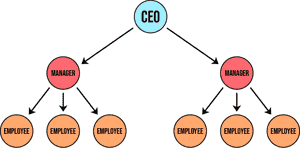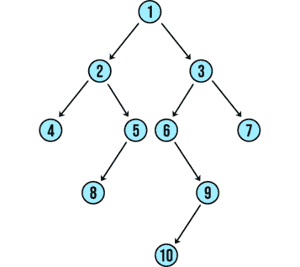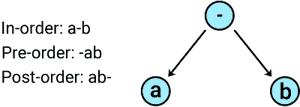Home/Blog/Tree traversal algorithms in Python every dev should know

# Tree traversal algorithms in Python every dev should know

Aug 28, 2022 - 8 min readAlgorithms are a significant part of a software development career, both in interviews and on the job. Many startups and FAANG companies like Facebook and Google focus majorly on algorithms and data structures during interviews, so understanding these concepts can be essential to advancing your career.

In addition, tech companies focus on designing the best algorithms to reduce server loading time, computational power, and so on, saving them a lot of time and resources. Therefore, it is important to understand how algorithms work and how they can be applied to specific computational tasks.

Today, we will discuss tree traversal algorithms in Python, which are commonly asked about during software engineering interviews.

We will specifically focus on Python over other programming languages due to its rising popularity among companies and easy-to-use syntax.

We’ll cover:

## What are trees?

In computer science terminology, trees are nonlinear hierarchical data structures.

A tree contains a collection of nodes (also called vertices) where a pair of nodes are connected to each other with an edge. Unlike a graph a tree has no cycles (so it’s called acyclic). We can see a tree in action using a hierarchical company structure below:Trees are not always the best choice of data structures, but in many instances, they lead to faster and memory-efficient algorithms and programs due to being non-linear in nature.A depiction of a tree data structure

## Tree nomenclature

• Node: A node, or vertex, is a structure that may contain data and connections to other nodes (known as its children). A link between two nodes is referred to as an edge. An edge could be outgoing or incoming to a node. For instance, in the figure above Node-2 has an incoming edge from Node-1 and two outgoing edges to Node-4 and Node-5. Leaf node: A node with no outgoing edges is referred to as a leaf node. In the above example, Node-4, Node-8, Node-10, and Node-7 are leaf nodes.

• R​​oot node: A root node has no incoming edges to it. Typically it is considered the topmost node of the tree. In our example, Node-1 is the root node. Typically, a tree must have precisely a single root node.

• Height of a node: The maximum number of edges from the node to a leaf node. In the above example, the heights of Node-3 and Node-4 are 3 and 0, respectively.

• Depth of a node: The number of edges from the root to nodes in a tree. In the above example, the depth of Node-3 and Node-10 are 1 and 4, respectively.

• Height of a tree: The height of the root node or depth of the deepest node.

• Out-degree and in-degree of a node: A node’s in-degree refers to the number of edges coming in, whereas out-degree refers to the number of outgoing edges. In the above example, Node-3 has the in-degree of 1 but the out-degree of 2.

• Pre-fix: This is where we access a tree starting from the root node first followed by the left child and then finally the right child.

• Post-fix: This is where we access a tree starting from the left child first, then right child, and finally the root node.

• In-fix: This is where we access a tree starting from the left child, the root node, and then finally the right child.

## How to traverse trees

Tree traversal is a process where we visit each and every node of a tree, optionally printing out data from those nodes. We always begin our search from the root node because all nodes are reachable from there.

There are two ways to traverse a tree. One is using the depth-first approach, and the second is using the breadth-first method.

#### Get hands-on with Ace the Python Coding Interview today.

Try one of our 400+ courses and learning paths: Ace the Python Coding Interview.

## Depth-first search

In depth-first search, we first go deep to a leaf node before visiting a sibling to a node. The depth-first approach is subdivided into the following categories:

• In-order traversal
• Pre-order traversal
• Post-order traversal

Let’s look at each of these three ways to traverse trees in Python. The following figure depicts in-order, pre-order, and post-order traversal for a simple tree.Figure depicting tree traversal

### In-order traversal

In this tree traversal method, we first visit the left sub-tree, then the root, and finally the right sub-tree.

Let’s look at in-order traversal in action. In the Python example below, we do the following:

• Line 5-9: Create a Node class. The class has three members
• Left child: contains the left child of the node
• Right child: holds the right child of the node
• Data: the value of the node
• Line 12-29: A function to insert data into the tree
• Line 31-43: A function to print the tree
• Line 46-54: Implementation of in-order traversal algorithm
• Line 56-65: Insert several nodes in the tree and print the result of an in-order traversal
from queue import Queue queue = Queue()class Node:   def __init__(self, val):        self.leftChild = None       self.rightChild = None       self.data = val  # Insert Node   def insert(self, data):       if data is None:           return       if self.leftChild is None:           self.leftChild = Node(data)           #print(self.data, '-- Left -->', data)           data = None       elif self.rightChild is None:                self.rightChild = Node(data)           #print(self.data, '-- Right -->', data)           data = None       else:           queue.put(self.leftChild)           queue.put(self.rightChild)        while queue.empty() is False:           queue.get().insert(data) # Print tree   def printTree(self):       ret = []       ret.append(self.data)       if self.leftChild is not None:           queue.put(self.leftChild)       if self.rightChild is not None:           queue.put(self.rightChild)        #print (len(stack))       while queue.empty() is False:           ret = ret + queue.get().printTree()        return ret  # Inorder traversal# leftChild -> parent -> rightChild   def inorderTraversal(self, root):       ret = []       if root:           ret = self.inorderTraversal(root.leftChild)           ret.append(root.data)           ret = ret + self.inorderTraversal(root.rightChild)       return ret root = Node(27)root.insert(14)root.insert(5)root.insert(10)root.insert(6)root.insert(31)root.insert(9)print("\n\nData is tree is = ", root.printTree()) print("\n\nresult of inorder traversal is = ", root.inorderTraversal(root))

### Pre-order traversal

When working with pre-order traversal, we visit the root node first, then the left sub-tree, and finally the right sub-tree.

In our example below, we will take the following steps:

• Line 4-9: Create a Node class. The class has three members
• Left child: contains the left child of the node
• Right child: contains the right child of the node
• Data: the value of the node
• Line 13-29: A function to insert data into the tree
• Line 32-43: A function to print tree
• Line 48-54: Implementation of pre-order traversal algorithm
• Line 56-65: Insert several nodes in the tree and print the result of the pre-order traversal
from queue import Queue queue = Queue()class Node:   def __init__(self, val):        self.leftChild = None       self.rightChild = None       self.data = val  # Insert Node   def insert(self, data):       if data is None:           return       if self.leftChild is None:           self.leftChild = Node(data)           #print(self.data, '-- Left -->', data)           data = None       elif self.rightChild is None:                self.rightChild = Node(data)           #print(self.data, '-- Right -->', data)           data = None       else:           queue.put(self.leftChild)           queue.put(self.rightChild)        while queue.empty() is False:           queue.get().insert(data) # Print tree   def printTree(self):       ret = []       ret.append(self.data)       if self.leftChild is not None:           queue.put(self.leftChild)       if self.rightChild is not None:           queue.put(self.rightChild)        #print (len(stack))       while queue.empty() is False:           ret = ret + queue.get().printTree()        return ret  # preorder traversal# parent -> leftChild -> rightChild   def preorderTraversal(self, root):       ret = []       if root:           ret.append(root.data)           ret = ret + self.preorderTraversal(root.leftChild)           ret = ret + self.preorderTraversal(root.rightChild)       return ret root = Node(27)root.insert(14)root.insert(5)root.insert(10)root.insert(6)root.insert(31)root.insert(9)print("\n\nData is tree is = ", root.printTree()) print("\n\nresult of preorder traversal is = ", root.preorderTraversal(root))

### Post-order traversal

As the name suggests, the post-order tree traversal is where we visit the root node last. In this traversal method, we traverse the left sub-tree first, followed by the right sub-tree, and finally the root.

Let’s learn by example. In the Python program below, we do the following:

• Line 4-9: Create a Node class. The class has three members
• Left child: contains the left child of the node
• Right child: contains the right child of the node
• Data: the value of the node
• Line 13-29: A function to insert data into the tree
• Line 31-43: A function to print tree
• Line 48-54: Implementation of post-order traversal algorithm
• Line 56-65: Insert several nodes in the tree and print the result of the post-order traversal
from queue import Queue queue = Queue()class Node:   def __init__(self, val):        self.leftChild = None       self.rightChild = None       self.data = val  # Insert Node   def insert(self, data):       if data is None:           return       if self.leftChild is None:           self.leftChild = Node(data)           #print(self.data, '-- Left -->', data)           data = None       elif self.rightChild is None:                self.rightChild = Node(data)           #print(self.data, '-- Right -->', data)           data = None       else:           queue.put(self.leftChild)           queue.put(self.rightChild)        while queue.empty() is False:           queue.get().insert(data) # Print tree   def printTree(self):       ret = []       ret.append(self.data)       if self.leftChild is not None:           queue.put(self.leftChild)       if self.rightChild is not None:           queue.put(self.rightChild)        #print (len(stack))       while queue.empty() is False:           ret = ret + queue.get().printTree()        return ret  # postorder traversal# leftChild -> rightChild -> parent   def postorderTraversal(self, root):       ret = []       if root:           ret = ret + self.postorderTraversal(root.leftChild)           ret = ret + self.postorderTraversal(root.rightChild)           ret.append(root.data)       return ret root = Node(27)root.insert(14)root.insert(5)root.insert(10)root.insert(6)root.insert(31)root.insert(9)print("\n\nData is tree is = ", root.printTree()) print("\n\nresult of postorder traversal is = ", root.postorderTraversal(root))

### Application of depth-first tree traversal algorithms

• In-order tree traversal method: this method is mostly used to obtain the non-decreasing order of nodes.
• Pre-order tree traversal method: this method is used to create tree copies and get tree prefix expressions.
• Post-order traversal method: we can use this method to delete and also to get postfix expressions of trees.

## Breadth-first search algorithms

Breadth-first search algorithms are commonly used on both trees and graphs. They’re implemented using recursion via data structures like lists and dictionaries.

The algorithms for breadth-first search (BFS) are as follows:

• Pick any node in the tree or graph. Enqueue all adjacent nodes into a queue. Dequeue a vertex, and mark it as visited. Enqueue all adjacent nodes into another queue.
• Repeat step 1 until all nodes have been visited or until you have arrived at your solution.
• Be sure to mark all nodes you go through as visited before proceeding to the next ones to avoid being stuck in an infinite loop.

In our interactive code editor above in the depth-first traversal codes, our function printTree is printing tree using BFS. We encourage you to carefully observe our implementation of printTree and play around with it.

## Master Python tree traversal algorithms today

Congratulations on completing your journey into Python tree traversal algorithms! But we’ve just touched the surface of what you can do with tree traversal algorithms, which also includes practicing for interviews.

Several algorithmic interview questions will require you to solve problems using a tree data structure. Some common interview questions are:

• Given a binary tree,
find its height
• Binary tree in-order traversal
• Maximum depth of a binary tree
• Sum root to leaf numbers

Additionally, you can check out the Educative learning path Ace the Python Coding Interview. You will learn how to handle all the nitty-gritty details of excelling at Python coding interviews, especially data structures and algorithms.

As always, happy learning!

### Continue learning about Python, data structures, and algorithms